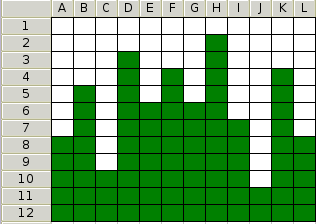# CS35 Lab3: Analysis

Due at the beginning of class Thursday, February 10

Run update35 to get copies of some useful files added to your cs35/labs/03 directory. You will not need to turn in any code this week. Instead you will turn in written solutions to the problems below as well as some graphs showing the results of various running time experiments.

You may work with a partner this week. However, you should work together on each problem rather than each working on only half of the problems. Make sure that both names are on the pages that you hand in.

Write solutions to the following problems
• C-3.14 An array A contains n-1 unique integers in the range 0 to n-1. Thus exactly one number in this range is missing in the array. Design an O(n) time algorithm for finding this missing number. However, you are only allowed O(1) extra space. You cannot make extra arrays of size n, but you can store a constant number of extra variables.
• Based on C-3.20 The image below shows an n by n grid that is stored in memory as a two dimensional array. The element at row i column j can be indexed in constant time using grid[i][j]. Each cell in the grid contains either a 1 or a 0 (indicated in the figure with green and white blocks). In any column, all the one's appear before any of the zeros. Given such a grid, design an O(n) algorithm for finding the column with the most ones (tallest green tower). Note there are O(n^2) cells, so you cannot check every cell in the grid.• Suppose I want to find the big-Oh runtime of a certain algorithm, but I do not have access to the description of the algorithm. All I know is that the runtime of the algorithm is given by the following recursive definition: T(n)=T(n-1)+O(n), meaning the time it takes to process an input of size n is equivalent to the time it takes to process an input of size n-1 plus a O(n) term. Assume T(0) is a constant. We could write T(1) <= T(0)+c*1 and T(2) <= T(1)+c*2 <= T(0)+c(1+2). Determine a big-Oh bound on the runtime of T(n) and prove your result using induction.
• Describe an algorithm for finding both the minimum and maximum of n numbers using fewer than 3n/2 comparisions. The following psuedocode finds the max using n-1 comparisions.
```input: Array A of size n>0
max=A
for(i=1; i<n; i++)
if(A[i] > max)
max=A[i]
```
Note I am only counting comparisions of the form A[i] < x. Don't count the comparisions in the loop (i<n).
• Give the best big-Oh bounds on the runtime of the following functions.
```Ex1(n)
for(i=0; i<n; i++)
a = i

Ex2(n)
for(i=0; i<n; i+=2)
a = i

Ex3(n)
for(i=0; i<n*n; i++)
a = i

Ex4(n)
for(i=0; i<n; i++)
for(j=0; j<=i; j++)
a = i

Ex5(n)
for(i=0; i<n*n; i++)
for(j=0; j<=i; j++)
a = i

Ex6(n)
k=1
for(i=0; i<n; i++)
for(j=0; j<k; j++)
a = j
k=k*2
```
Running time experiments

Perform an experimental analysis that compares the relative running times of the functions you considered in Ex1 through Ex6 above.

In running these experiments, you may need to use very different sizes of n, depending on the example being tested. Fast algorithms may not start showing any distinctions until n gets to be greater than 100,000. Slower algorithms may begin showing differences when n is nearer to 100. One really slow algorithm might not be able to process n=100 in the lifetime of the universe.

`pgraph ex4results`
`lpr mygraph.ps`